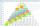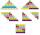# AP RT triangle

The length of the sides of a right triangle form an arithmetic progression, longer leg is 24 cm long. What are the perimeter and area?

Result

o =  72 cm
S =  216 cm2

#### Solution:Leave us a comment of example and its solution (i.e. if it is still somewhat unclear...):

Showing 0 comments:Be the first to comment!#### To solve this example are needed these knowledge from mathematics:

Do you have a linear equation or system of equations and looking for its solution? Or do you have quadratic equation? Pythagorean theorem is the base for the right triangle calculator.

## Next similar examples:

1. RT leg and perimeterCalculate the length of the sides of a right triangle ABC with hypotenuse c when the length of a leg a= 84 and perimeter of the triangle o = 269.
2. Right angled triangleHypotenuse of a right triangle is 17 cm long. When we decrease length of legs by 3 cm then decrease its hypotenuse by 4 cm. Determine the size of legs.
3. RTriangle 17The hypotenuse of a right triangle is 17 cm. If you decrease both two legs by 3 cm you will reduce the hypotenuse by 4 cm. Determine the length of this legs.
4. CathetiThe hypotenuse of a right triangle is 41 and the sum of legs is 49. Calculate the length of its legs.
5. Circle annulusThere are 2 concentric circles in the figure. Chord of larger circle 10 cm long is tangent to the smaller circle. What are does annulus have?
6. Third memberDetermine the third member of the AP if a4=93, d=7.5.
7. Difference AP 4Calculate the difference of the AP if a1 = 0.5, a2 + a3 = -1.1
8. Arithmetic progressionIn some AP applies: 5a2 + 7a5 = 90 s3 = 12 Find the first member a =? and difference d = ?
9. Determine APDetermine the difference of the arithmetic progression if a3 = 7, and a4 + a5 = 71
10. AP - basicsDetermine first member and differentiate of the the following sequence: a3-a5=24 a4-2a5=61
11. AS sequenceIn an arithmetic sequence is given the difference d = -3 and a71 = 455. a) Determine the value of a62 b) Determine the sum of 71 members.
12. SeatsSeats in the sport hall are organized so that each subsequent row has five more seats. First has 10 seats. How many seats are: a) in the eighth row b) in the eighteenth row
13. SequenceBetween numbers 1 and 53 insert n members of the arithmetic sequence that its sum is 702.
14. Sequence 3Write the first 5 members of an arithmetic sequence: a4=-35, a11=-105.
15. Ball gameRichard, Denis and Denise together scored 932 goals. Denis scored 4 goals over Denise but Denis scored 24 goals less than Richard. Determine the number of goals for each player.
16. Linsys2Solve two equations with two unknowns: 400x+120y=147.2 350x+200y=144
17. Theorem proveWe want to prove the sentense: If the natural number n is divisible by six, then n is divisible by three. From what assumption we started?